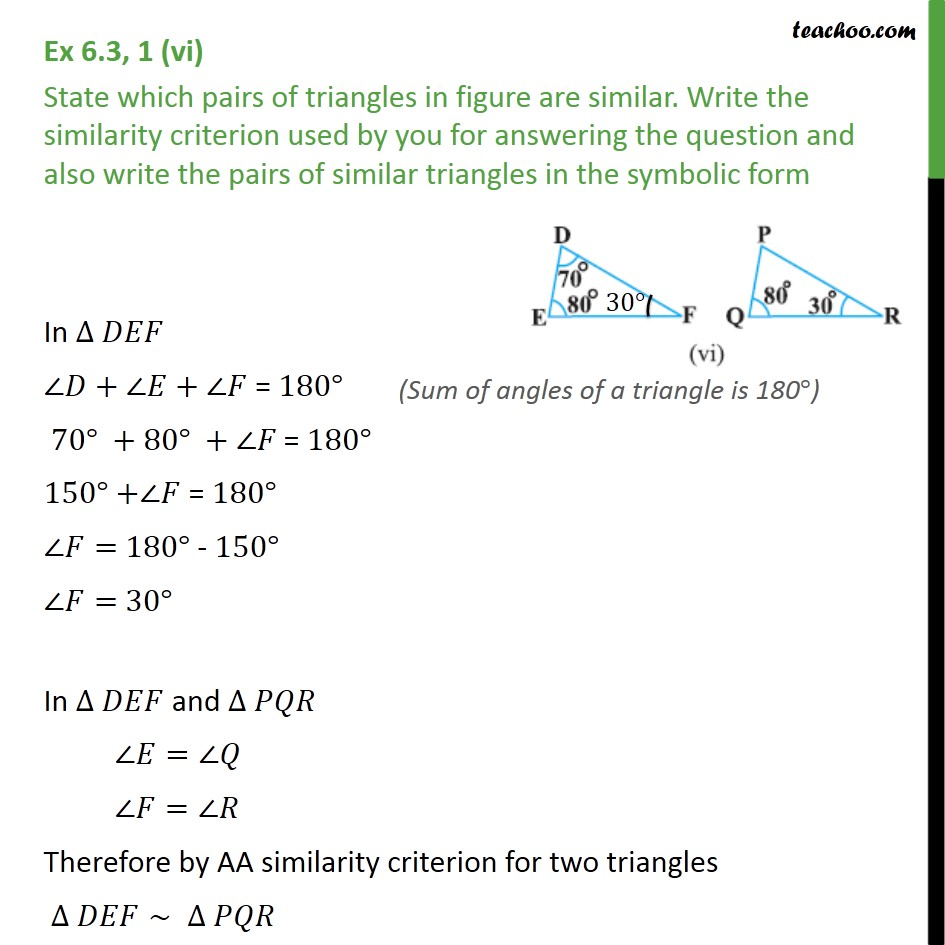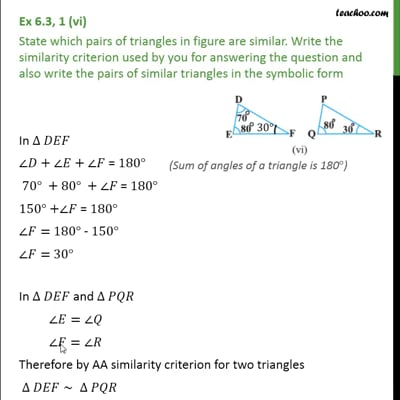Ex 6.3

Chapter 6 Class 10 Triangles (Term 1)
Serial order wiseThis video is only available for Teachoo black users

### Transcript

Ex 6.3, 1 (vi) State which pairs of triangles in figure are similar. Write the similarity criterion used by you for answering the question and also write the pairs of similar triangles in the symbolic form In + + = 180 70 " "+80 " "+ " = " 180 150 + = 180 = 180 - 150 = 30 In and = = Therefore by AA similarity criterion for two triangles " "